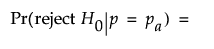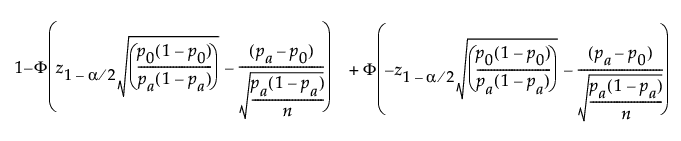Publication date: 05/24/2021

## Power for One Sample Proportion

Use the Power Explorer for One Sample Proportion to determine a sample size for a hypothesis test about one proportion. Select DOE > Sample Size Explorers > Power > Power for One Sample Proportion. Explore the trade offs between sample size, power, significance, and the hypothesized difference to detect. Sample size and power are associated with the following hypothesis test: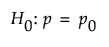versus the two-sided alternative: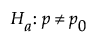or versus a one-sided alternative: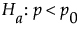or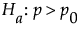where p is the population proportion and p0 is the null proportion.

### Power Explorer for One Sample Proportion Options

Set study assumptions and explore sample sizes using the radio buttons, text boxes, and sliders. The curve updates as you make changes to the settings. Alternatively, change settings by dragging the cross hairs on the curve or adjusting the values in the axis text boxes.

Test Type

Specifies a one or two-sided hypothesis test.

Model Type

Exact Test

Specifies calculations based on the Clopper-Pearson methodology.

Normal Approximation

Specifies calculations based on the normal approximation methodology.

Tip: Since the binomial distribution is discrete, the actual test size can differ significantly from the stated Alpha level for small samples or proportions near 0 or 1. To guarantee an alpha level equal to or greater than your stated level, use the Exact test.

Fixed Parameters

Alpha

Specifies the probability of a type I error, which is the probability of rejecting the null hypothesis when it is true. It is commonly referred to as the significance level of the test. The default alpha level is 0.05.

Test Parameters

Parameters that are inter-related and update as you make changes.

Assumed proportion (p0)

Specifies the proportion that you anticipate or assume for your study, the null hypothesis value.

Alternative proportion (pA)

Specifies the proportion that you test against, the alternative hypothesis value.

Sample Size

Specifies the total number of observations (runs, experimental units, or samples) needed for your experiment.

Power

Specifies the probability of rejecting the null hypothesis when it is false. With all other parameters fixed, power increases as sample size increases.

Save Settings

Saves the current settings to the Saved Settings table. This enables you to save a set of alternative study plans. See Saved Settings in the Sample Size Explorers.

Make Data Collection Table

Creates a new data table that you can use for data collection.

### Statistical Details for the One Sample Proportion Explorer

For the exact test, the power is computed based on the form of the alternative hypothesis.

For one-sided, higher alternative: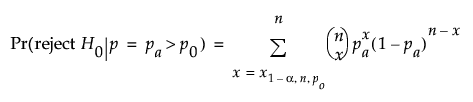for a one-sided, lower alternative: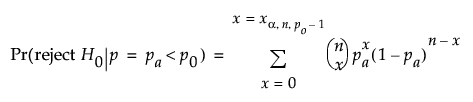for a two-sided alternative: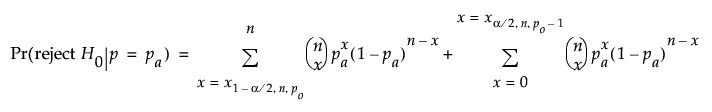where xq,n,p is the qth quantile of a binomial distribution with n trials and probability p.

For the normal approximation, the power is computed based on the form of the alternative hypothesis.

For a one-sided, higher alternative: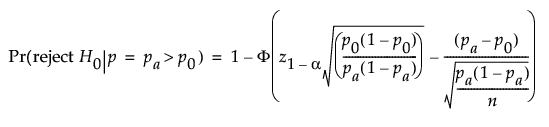For a one-sided, lower alternative: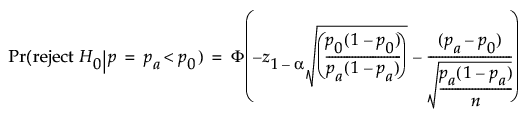For a two-sided alternative: